Question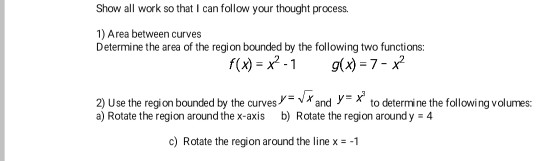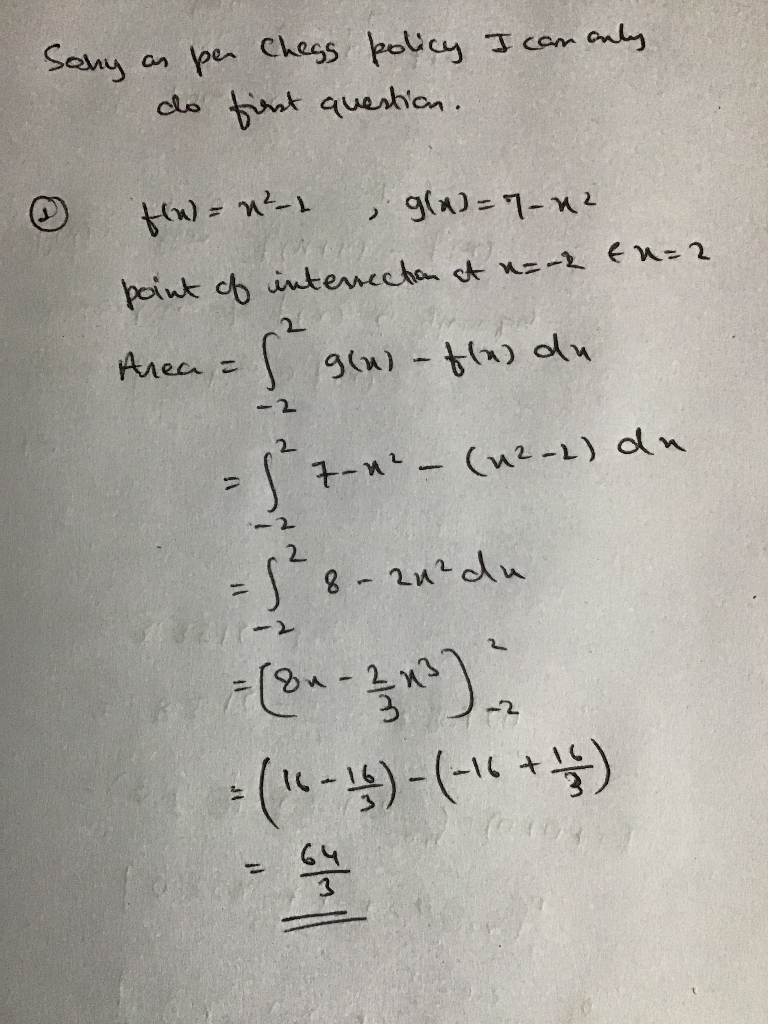#### Earn Coins

Coins can be redeemed for fabulous gifts.

Similar Homework Help Questions
• ### Show all work please. 1Find the area of the region bounded by the curves y 2x2...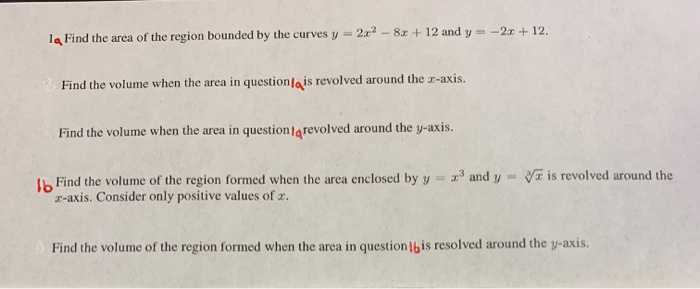Show all work please. 1Find the area of the region bounded by the curves y 2x2 - 8x + 12 and y=-2 + 12. Find the volume when the area in question/qis revolved around the 3-axis. Find the volume when the area in question/grevolved around the y-axis. Va is revolved around the Ib Find the volume of the region formed when the area enclosed by y = x3 and y 2-axis. Consider only positive values of x. Find the volume...

• ### show all work 1. Find the area of the region bounded by the curves below. Sketch...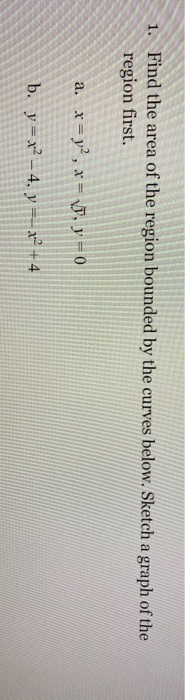show all work 1. Find the area of the region bounded by the curves below. Sketch a graph of the region first. a. x = y2, x = VD, y = 0 b. y = x2 – 4, y == x2 + 4

• ### 16 pts) 1. Determine the area of the region between the two curves y=x and y+2x...16 pts) 1. Determine the area of the region between the two curves y=x and y+2x by integrating over the x-axis. Hint: Refer the figure and note that you will have two integrals to solve by splitting the region between the two curves into two smaller regions. lo pl [6 pts) 2. Find the area of the region bounded by the curves y=12 - x, y=vx, and y20

• ### Find the area of the region between curves       1. Find Find the area of...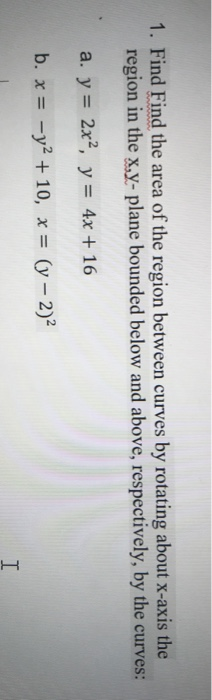Find the area of the region between curves       1. Find Find the area of the region between curves by rotating about x-axis the region in the x,y- plane bounded below and above, respectively, by the curves: a. y = 2x2, y = 4x + 16 b. x = -y2 + 10, x = (y – 2) I

• ### show all steps thx 6. Find the area of the region bounded between the curves y...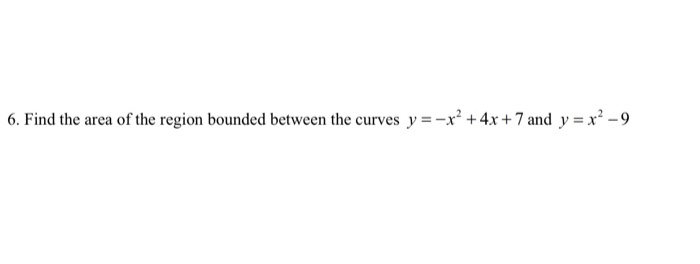show all steps thx 6. Find the area of the region bounded between the curves y = -x² + 4x + 7 and y = x² - 9

• ### 2. Graph the following equations and shade the area of the region between two curves. Determine...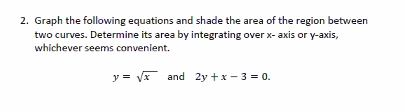2. Graph the following equations and shade the area of the region between two curves. Determine its area by integrating over x-axis or y-axis, whichever seems convenient. y = v* and 2y + x 3 = 0.

• ### 1) Find the exact area bounded between the sine and cosine functions on the interval 4' 4 Clearly show your work in...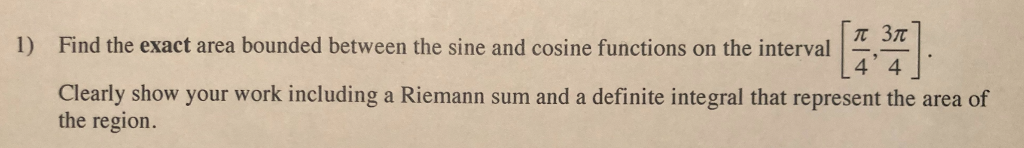1) Find the exact area bounded between the sine and cosine functions on the interval 4' 4 Clearly show your work including a Riemann sum and a definite integral that represent the area of the region. 1) Find the exact area bounded between the sine and cosine functions on the interval 4' 4 Clearly show your work including a Riemann sum and a definite integral that represent the area of the region.

• ### 1. Determine the area of the region between the two curves y=x' and y = x...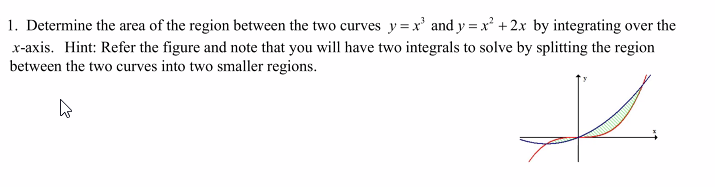1. Determine the area of the region between the two curves y=x' and y = x + 2x by integrating over the x-axis. Hint: Refer the figure and note that you will have two integrals to solve by splitting the region between the two curves into two smaller regions. W t.

• ### Home Work Task 1 Total M Determine the area of the region enclosed by the two...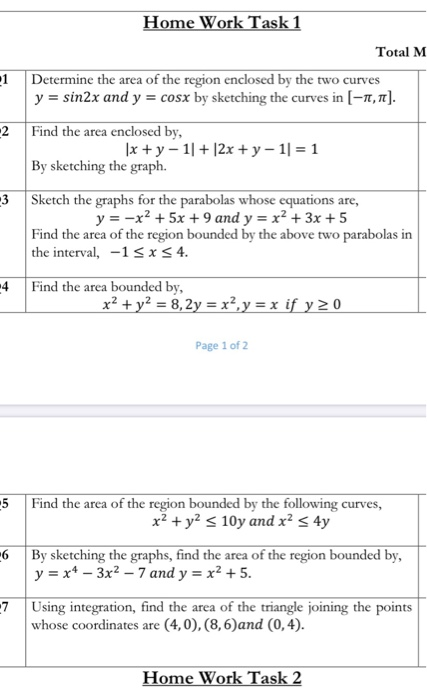Home Work Task 1 Total M Determine the area of the region enclosed by the two curves y = sin2x and y = cosx by sketching the curves in (-1,1]. Find the area enclosed by, 1x + y - 11 + 12x + y - 11 = 1 By sketching the graph. 3 Sketch the graphs for the parabolas whose equations are, y = -x2 + 5x + 9 and y = x² + 3x + 5 Find the area...

• ### Please show all work 1. Find the area of the region bounded by the graphs of...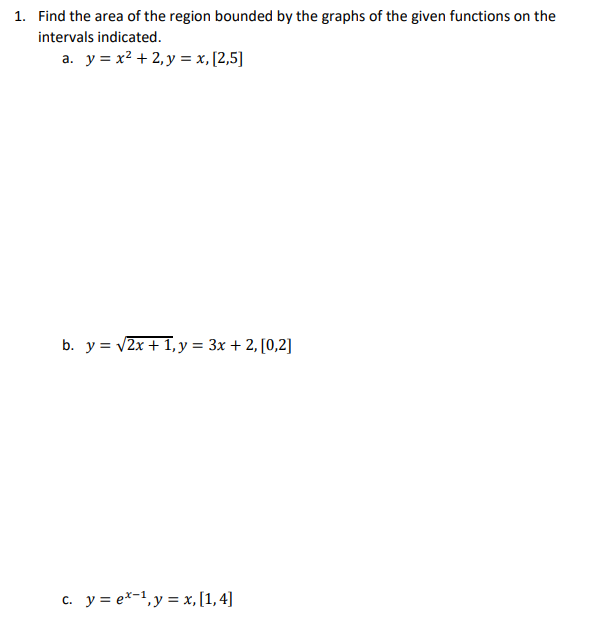Please show all work 1. Find the area of the region bounded by the graphs of the given functions on the intervals indicated. a. y = x2 + 2, y = x, (2,5) b. y = (2x +1, y = 3x + 2, [0,2] C. y = ex-1, y = x,[1,4]